Courses

# Test: Current Electricity Level - 2

## 30 Questions MCQ Test Exclusive Video Lectures of Class 12 Physics by Experts | Test: Current Electricity Level - 2

Description
This mock test of Test: Current Electricity Level - 2 for Class 12 helps you for every Class 12 entrance exam. This contains 30 Multiple Choice Questions for Class 12 Test: Current Electricity Level - 2 (mcq) to study with solutions a complete question bank. The solved questions answers in this Test: Current Electricity Level - 2 quiz give you a good mix of easy questions and tough questions. Class 12 students definitely take this Test: Current Electricity Level - 2 exercise for a better result in the exam. You can find other Test: Current Electricity Level - 2 extra questions, long questions & short questions for Class 12 on EduRev as well by searching above.
QUESTION: 1

Solution:
QUESTION: 2

Solution:
QUESTION: 3

### A current (I) flows through a uniform wire of diameter (d) when the mean drift velocity is V. The same current will flow through a wire of diameter d/2 made of the same material if the mean drift velocity of the electron is

Solution: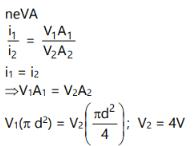QUESTION: 4

When a potential difference (V) is applied across a conductor , the thermal speed of electrons is

Solution:
QUESTION: 5

A steady current is passing through a linear conductor of non-uniform cross-section. The current density in the conductor is

Solution:
QUESTION: 6

A metallic block has no potential difference applied across it. Then the mean velocity of free electron is

Solution:
QUESTION: 7

The resistance of some substances become zero at very low temperature , then these substances are called

Solution:
QUESTION: 8

The resistance of wire is 20W. The wire is stretched to three times its length. Then the resistance will now be

Solution:

Unit of resistance should be 20ohm

Solution :- Resistance, R= ρl/A

When length l increases three times, the cross section A will decease three times of previous. So length becomes 3l and cross section becomes A/3.

Now resistance becomes ,R  = ρ3l / πA/3

​ =9R=9×20=180Ω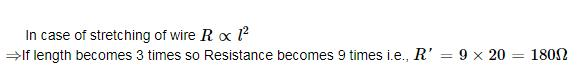QUESTION: 9

The resistance of a semi-conductors

Solution:
QUESTION: 10

A hollow copper tube of 5 m length has got external diameter equal to 10 cm and the walls are 5 mm thick. If specific resistance of copper is 1.7 × 10-8 ohm × metre. calculate the resistance of the tube.

Solution:
QUESTION: 11

In order to increase the resistance of a given wire of uniform cross section to four times its value, a fraction of its length is stretched uniformly till the full length of the wire becomes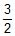times the original length what is the value of this fraction ?

Solution:

Let, the length of wire = l unit

Fraction required =a

Then, the lenght of wire which is stretched =al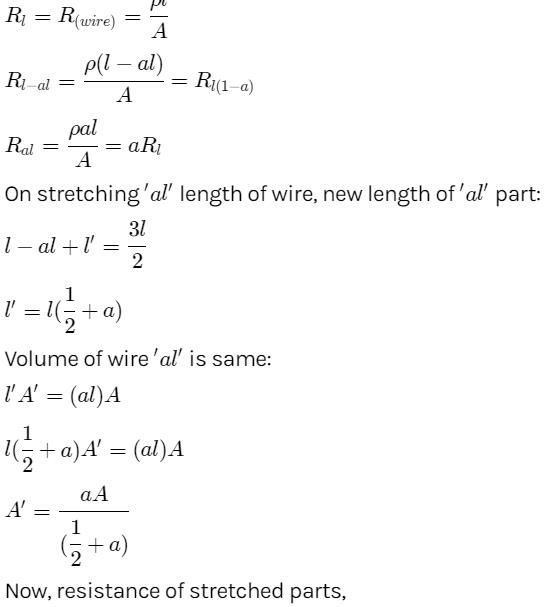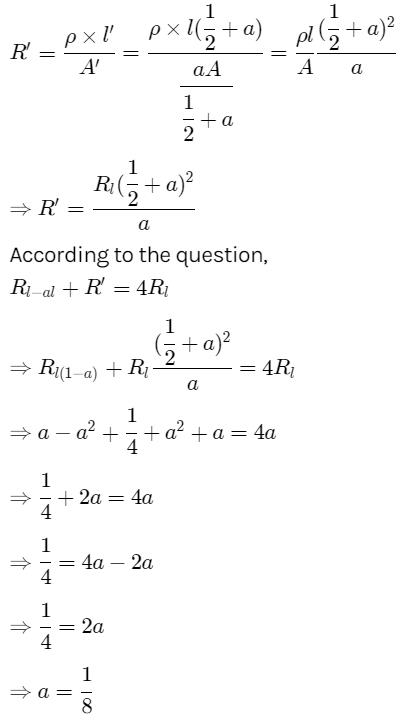QUESTION: 12

A conductor with rectangular cross section has dimensions (a × 2a × 4a) as shown in figure. Resistance across AB is x, across CD is y and across EF is z. Then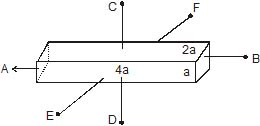Solution:
QUESTION: 13

A negligibly small current is passed through a wire of length 15 m and uniform cross-section 6.0×10-7 m2. The measured resistance of the wire is 5.0 W. The resistivity of the material is

Solution:
QUESTION: 14

The resistance of a wire of length 20 cm is 5W. It is stretched uniformly to a length of 40 cm. The resistance now becomes:

Solution:

Unit of resistance should be 5ohm.

Resistance of a wire is given by the formula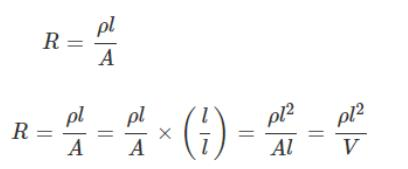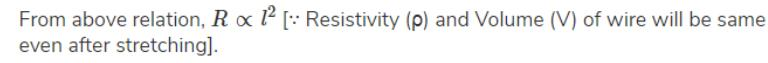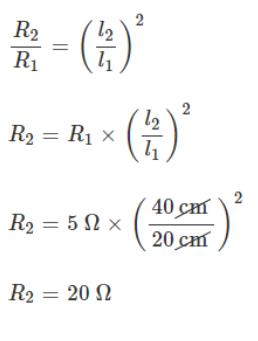QUESTION: 15

Two wires each of radius of cross section r but of different materials are connected together end to end (in series). If the densities of charge carriers in the two wires are in the ratio 1 : 4, the drift velocity of electrons in the two wires will be in the ratio.

Solution:
QUESTION: 16

In a wire of cross-section radius r, free electrons travel with drift velocity v when a current I flows through the wire. What is the current in another wire of half the radius and of the same material when the drift velocity is 2v ?

Solution:

Here we are using the formula
current = neAv ...............(1)
where n= number of electron density
A =area of cross section
e= charge of electron
v=drift velocity
As both the wires are of the same materials then the electron density is the same for both.
For the first wire, current I is given by,
I = neAv
Now according to the problem another wire of half the radius and of the same material when the drift velocity is 2v
Therefore for the second wire current is = ne(A/4)(2v)
= (neAv)/2
now putting I at this place
= I/2

QUESTION: 17

Read the following statements carefully :

Y : The resistivity of a semiconductor decreases with increases of temperature.

Z : In a conducting solid, the rate of collision between free electrons and ions increases with increase of temperature.

Select the correct statement from the following

Solution:
QUESTION: 18

A piece of copper and another of germanium are cooled from room temperature to 80K. The resistance of

Solution:
QUESTION: 19

An insulating pipe of cross-section area `A' contains an electrolyte which has two types of ions ® their charges being _e and +2e. A potential difference applied between the ends of the pipe result in the drifting of the two types of ions, having drift speed = v (_ve ion) and v/4 (+ ve ion). Both ions have the same number per unit volume = n. The current flowing through the pipe is

Solution:
QUESTION: 20

Two wires of the same material having radii in the ratio 1:2, carry currents in the ratio 4 : 1. The ratio of drift velocities of electrons in them is

Solution:

We have the relation between current I, n = no. of carrier per unit volume, drift velocity ve and CSA A as.

I = neAv

Let current flowing in the 2nd wire I2 = I thus current flowing through the 1st wire I1 = 4I

Let the radius of the first wire be r thus radius of the 2nd wire = 2r

In the first wire

A1 = πr2

In the 2nd wire

A2 = π(2r)2 = 4πr2

I1/ I2 = (A1v1 )/(A2v2 )  (ne being same for two wires as they are made of the same material)

4I/I = πr2 v1/4 πr2v2

=> v1/v2 = 16/1

=> v­1:v2 = 16:1

Thus ratio of the drift velocities are 16:1

QUESTION: 21

A current I flows through a uniform wire of diameter d when the mean electron drift velocity is v. the same current will flow through a wire of diameter d/2 made of the same material if the mean drift velocity of the electron is

Solution: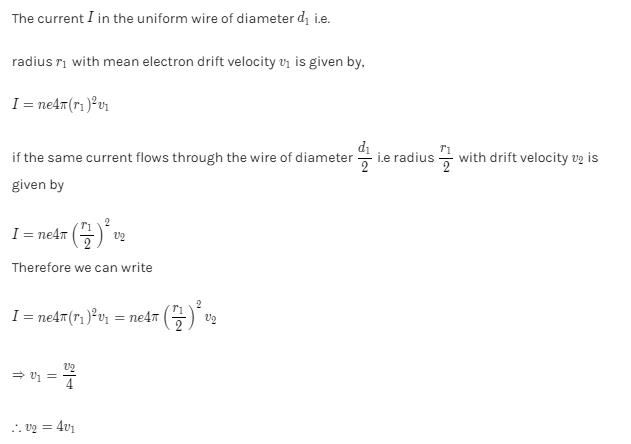QUESTION: 22

The current in a metallic conductor is plotted against voltage at two different temperatures T1 and T2. Which is correct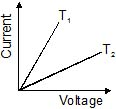Solution:
QUESTION: 23

A uniform copper wire carries a current i amperes and has p carriers per metre3. The length of the wire is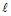metres and its cross-section area is s metre2. If the charge on a carrier is q coulombs, the drift velocity in ms-1 is given by

Solution:
QUESTION: 24

In the presence of an applied electric field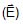in a metallic conductor.

Solution:
QUESTION: 25

A wire has a non-uniform cross-section as shown in figure. A steady current flows through it. The drift speed of electrons at points P and q is vp and vQ.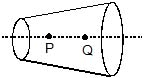Solution:
QUESTION: 26

A rectangular carbon block has dimensions 1.0 cm × 1.0 cm × 50 cm. Resistances are measured, first across two square ends and then across two rectangular ends, respectively. If resistivity of carbon is 3.5 × 10_5 W-m, then values of measured resistances respectively are :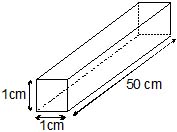Solution:
QUESTION: 27

A storage battery is connected to a charger for charging with a voltage of 12.5 Volts. The internal resistance of the storage battery is 1 W. When the charging current is 0.5 A, the emf of the storage battery is :

Solution:
QUESTION: 28

The terminal voltage across a battery of emf E can be

Solution:
QUESTION: 29

In order to determine the e.m.f of a storage battery it was connected in series with a standard cell in a certain circuit and a current I1 was obtained. When the battery is connected to the same circuit opposite to the standard cell a current I2 flow in the external circuit from the positive pole of the storage battery was obtained. What is the e.m.f e1 of the storage battery? The e.m.f of the standard cell is e2.

Solution:
QUESTION: 30

One end of a Nichrome wire of length 2L and cross-sectional area A is attatched to an end of another Nichrome wire of length L and cross-sectional area 2A. If the free end of the longer wire is at an electric potential of 8.0 volts, and the free end of the shorter wire is at an electric potential of 1.0 volt, the potential at the junction of the two wires is equal to

Solution: